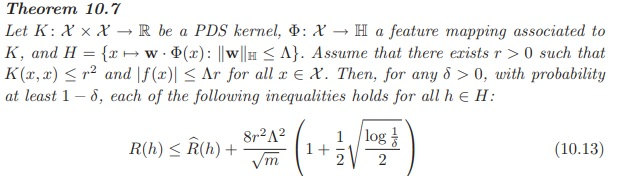# #StackBounty: #cross-validation #ridge-regression #vc-dimension Rademacher Bound, An Alternative to Cross Validation for Ridge?

### Bounty: 100

Below is a theorem from the book “Foundations of Machine Learning”.It specifies the generalization bounds for Kernel Ridge Regression by making use of the Rademacher Complexity on linear models. $$R(h)$$ is the generalization error, and $$hat{R}(h)$$ is the empirical error. Now pretty much everything is either known to us, picked by us, or can be calculated by us. $$m$$ is the number of training samples.

Instead of finding the right penalty $$Lambda$$ via cross validation, can we simply pick the $$Lambda$$ that minimizes the right hand side of the inequality? What should be the $$delta$$ value to be set in order to achieve best predictive result? How to choose $$r$$ as tight as possible?

Is this an alternative to Cross Validation for Kernel Ridge (or just Ridge) Regression?

Get this bounty!!!

This site uses Akismet to reduce spam. Learn how your comment data is processed.